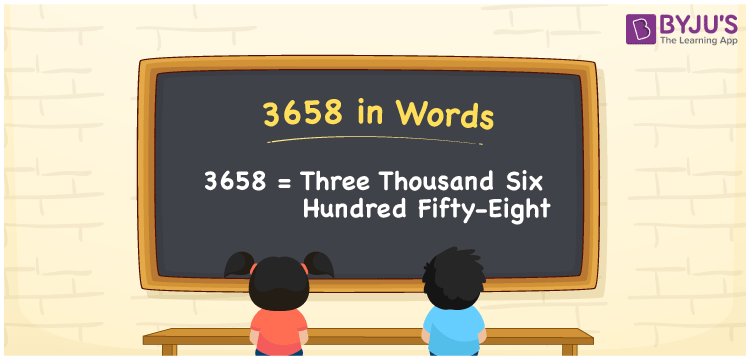# 3658 in Words

We can write 3658 in words as Three Thousand Six Hundred Fifty-eight. Suppose you bought a new fan that costs Rs. 3658, then you could say that “ I bought a new fan for Three Thousand Six Hundred Fifty-eight rupees”. In this article, you will learn how to convert the number 3658 into words in an exciting way. The number 3658 is used in expressions that relate to money, days, distance, length, weight and so on.

 3658 in words Three Thousand Six Hundred Fifty-eight Three Thousand Six Hundred Fifty-eight in Numbers 3658

## 3658 in English Words

We generally write numbers in words using the English alphabet. Thus, we can read 3658 in English as “Three Thousand Six Hundred Fifty-eight”.## How to Write 3658 in Words?

In this section, you will learn how to write the number 3658 in words. The given number 3658 has four digits, so we should make a place value chart that represents the place value for all these four digits. The order of place values of digits in a number is in accordance with the Indian numbering system, such as:

• Ones
• Tens
• Hundreds
• Thousands
• Ten-thousands
• Hundred-thousands or Lakhs

This can be done as follows.

 Thousands Hundreds Tens Ones 3 6 5 8

Thus, we can write the expanded form as:

3 × Thousand + 6 × Hundred + 5 × Ten + 8 × One

= 3 × 1000 + 6 × 100 + 5 × 10 + 8 × 1

= 3658

= One thousand five hundred

3658 is a natural number that precedes 3659 and succeeds 3657.

3658 in words – Three Thousand Six Hundred Fifty-eight

Is 3658 an odd number? – No

Is 3658 an even number? – Yes

Is 3658 a perfect square number? – No

Is 3658 a perfect cube number? – No

Is 3658 a prime number? – No

Is 3658 a composite number? – Yes

## Related Articles

75000 in words = Seventy-five thousand

## Frequently Asked Questions on 3658 in Words

Q1

### How do you write 3658 in English?

We can write 3658 in English words as “Three Thousand Six Hundred Fifty-eight”.
Q2

### How do you write 3658 in words for a cheque?

For a cheque, we generally write 3658 in words as Three Thousand Six Hundred Fifty-eight rupees only.
Q3

### What number is 3658 in words?

The number 3658 is Three Thousand Six Hundred Fifty-eight in words.